# GRE Subject Test: Math : Range & IQR

## Example Questions

← Previous 1

### Example Question #1 : Range & Iqr

What is the range of the set of numbers: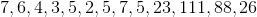?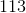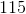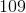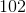Explanation:

Step 1: Arrange the numbers in the set from smallest to largest:

After we arrange, we get: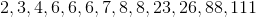.

Step 2: To find the range, subtract the largest number from the smallest number.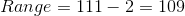The range of the data set is.

### Example Question #2 : Range & Iqr

What is the range of the numbers: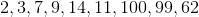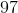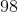Explanation:

Step 1: Re-write all numbers in order from smallest to largest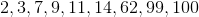.

Step 2: Subtract the largest number and smallest number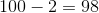The range is.

### Example Question #31 : Probability & Statistics

What is the range of the set: {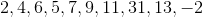}Explanation:

Step 1: Arrange the numbers from smallest to largest:

We get: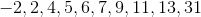Step 2: Find the range.. To find this, subtract the biggest number from the smallest number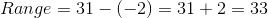The range is### Example Question #4 : Range & Iqr

Find the range of the set of numbers: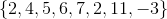Explanation:

Step 1: Arrange the numbers from smallest to largest. If the numbers are already written that way, then skip this step..

The numbers need to be arranged.. We will get: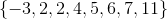Step 2: To find the range, subtract the smallest number from the biggest number...

So,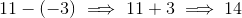The range of this set of numbers is...

### Example Question #1 : Range & Iqr

What is the range of the numbers: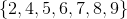Explanation:

Step 1: See if the numbers are in order..

The numbers are in order from smallest to largest..

Step 2: Subtract the largest number and the smaller number: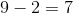is the range.

### Example Question #1 : Range & Iqr

What is the range of: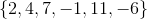Explanation:

Step 1: Rearrange the numbers from smallest to largest:

After rearranging, we have: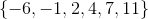Step 2: Find the smallest number:

The smallest number is.

Step 3: Find the largest number:

The largest number is.

Step 4: Subtract the smallest number from the smallest number: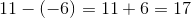The range is.

### Example Question #7 : Range & Iqr

Find the range of: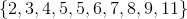Explanation:

Step 1: Locate the smallest and largest numbers in the set above...

Largest is, smallest isStep 2: To find the range, subtract smallest from largest...

Range=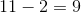### Example Question #1 : Range & Iqr

What is the range of the set,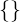?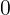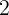Does Not Exist

Does Not Exist

Explanation:

Step 1: Define Range of a set..

You can calculate the range by subtracting the smallest number from the largest number in the set..

Step 2: There are no numbers in this set. This set is also known as the empty set..

The range of a set that has no elements does not exist.

### Example Question #1 : Range & Iqr

If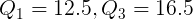, find the IQR (Inter-Quartile Range)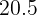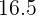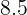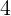Explanation:

Step 1: To find the Inter-Quartile Range, we need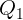and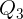.

The formula for the Inter-Quartile Range is: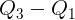.

Step 2: Plug in the given values of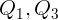to the equation...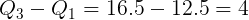So, the IQR=### Example Question #1 : Range & Iqr

Find the range: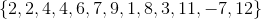Explanation:

Rearrange the numbers in the set from smallest to largest: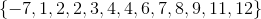To find the range, subtract the smallest number from the bigger number: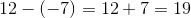The range is.

← Previous 1

### All GRE Subject Test: Math Resources